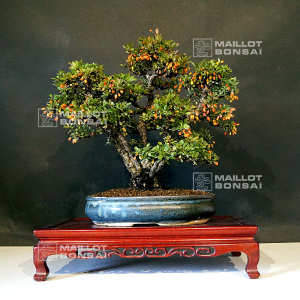##### The Japanese Bonsai specialist
Direct order Contact Help / Services Newsletter# Berberis thunbergii ref 160402114

Berberis thunbergiiref. : 10966Product unavailable

###### Description

Height of the only tree : 360 mm.

Width of the branches : 450 mm.
Dimensions of the old enamelled pot : 320*215*70 mm.

5-trunk kabudachi style, formed from layering with ramified trays. The bark is formed because this bonsaï is more than 25 years old.

Superb bonsaï from Kawahara nursery in Kawasaki. Golden yellow flowers in April, deciduous green foliage in summer and then red orange to golden yellow in fall.

This tree was repoted in March 2021 in pure akadama soil. This type of bonsaï requires a wintering protected from strong frost. It is rare, even in Japan, to see berberis bonsaï but it has many attractives traits : its yellow hanging flowers, its little round leaves and its beautiful fall colors. It will make the hapinness of a bonsaï lover.

Not any strong wire or cutting wound.

Shelf not included. Photographed without leaves in April 2021 and with leaves in May 2021.

#bonsai 7.6 #berberis 3.8 #yellow 3.6 #leaves 3.6 #this 3.4 #from 3.4 #thunbergii 3 #flowers 2.7 #outdoor 2.7 #strong 2.6

Formule
(( ROUND((CHAR_LENGTH(b.article_nom)-CHAR_LENGTH(REPLACE(b.article_nom, 'bonsai', '')))/LENGTH('bonsai')) + ROUND((CHAR_LENGTH(b.article_description)-CHAR_LENGTH(REPLACE(b.article_description, 'bonsai', '')))/LENGTH('bonsai')) ) * 5.6) + (( ROUND((CHAR_LENGTH(b.article_nom)-CHAR_LENGTH(REPLACE(b.article_nom, 'berberis', '')))/LENGTH('berberis')) + ROUND((CHAR_LENGTH(b.article_description)-CHAR_LENGTH(REPLACE(b.article_description, 'berberis', '')))/LENGTH('berberis')) ) * 3.8) + (( ROUND((CHAR_LENGTH(b.article_nom)-CHAR_LENGTH(REPLACE(b.article_nom, 'yellow', '')))/LENGTH('yellow')) + ROUND((CHAR_LENGTH(b.article_description)-CHAR_LENGTH(REPLACE(b.article_description, 'yellow', '')))/LENGTH('yellow')) ) * 3.6) + (( ROUND((CHAR_LENGTH(b.article_nom)-CHAR_LENGTH(REPLACE(b.article_nom, 'leaves', '')))/LENGTH('leaves')) + ROUND((CHAR_LENGTH(b.article_description)-CHAR_LENGTH(REPLACE(b.article_description, 'leaves', '')))/LENGTH('leaves')) ) * 3.6) + (( ROUND((CHAR_LENGTH(b.article_nom)-CHAR_LENGTH(REPLACE(b.article_nom, 'from', '')))/LENGTH('from')) + ROUND((CHAR_LENGTH(b.article_description)-CHAR_LENGTH(REPLACE(b.article_description, 'from', '')))/LENGTH('from')) ) * 3.4) + (( ROUND((CHAR_LENGTH(b.article_nom)-CHAR_LENGTH(REPLACE(b.article_nom, 'this', '')))/LENGTH('this')) + ROUND((CHAR_LENGTH(b.article_description)-CHAR_LENGTH(REPLACE(b.article_description, 'this', '')))/LENGTH('this')) ) * 3.4) + (( ROUND((CHAR_LENGTH(b.article_nom)-CHAR_LENGTH(REPLACE(b.article_nom, 'thunbergii', '')))/LENGTH('thunbergii')) + ROUND((CHAR_LENGTH(b.article_description)-CHAR_LENGTH(REPLACE(b.article_description, 'thunbergii', '')))/LENGTH('thunbergii')) ) * 3) + (( ROUND((CHAR_LENGTH(b.article_nom)-CHAR_LENGTH(REPLACE(b.article_nom, 'flowers', '')))/LENGTH('flowers')) + ROUND((CHAR_LENGTH(b.article_description)-CHAR_LENGTH(REPLACE(b.article_description, 'flowers', '')))/LENGTH('flowers')) ) * 2.7) + (( ROUND((CHAR_LENGTH(b.article_nom)-CHAR_LENGTH(REPLACE(b.article_nom, 'strong', '')))/LENGTH('strong')) + ROUND((CHAR_LENGTH(b.article_description)-CHAR_LENGTH(REPLACE(b.article_description, 'strong', '')))/LENGTH('strong')) ) * 2.6) + (( ROUND((CHAR_LENGTH(b.article_nom)-CHAR_LENGTH(REPLACE(b.article_nom, 'golden', '')))/LENGTH('golden')) + ROUND((CHAR_LENGTH(b.article_description)-CHAR_LENGTH(REPLACE(b.article_description, 'golden', '')))/LENGTH('golden')) ) * 2.6)

## Secure payment## Delivery

Our logistic partners :04 74 55 23 48
Pépinière MAILLOT-BONSAÏ
Le Bois Frazy
01990 RELEVANT - FRANCE
on appointment